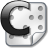# Recursive Descent Parser

## Recursive Descent Parser in C

The recursive descent parser is the core module of the calculator application found on the COMA, the XMultiKit and the Xmegalab. It is based on the Visual Basic program Math Expression Evaluator by Michael Combs.

I ported the code to standard C so it can be used on any platform.

To use this module you only need to add parse.c to your project and #include "parse.h" in your code.

Two global variables are used:

extern uint8_t m_error; // different than zero if an error occurred
extern double ans;      // answer

The only function you need to call is

void evaluate(char expression[]); // the expression must end with a space

The function will modify the global variables m_error and ans.

Example:

evaluate("1+1 "); // The expresion must and with a space

ans will equal 2, m_error will equal 0.

Download the files here:

###Recursive Descent Parser

Here is a calculator demo using the recursive descent parser: